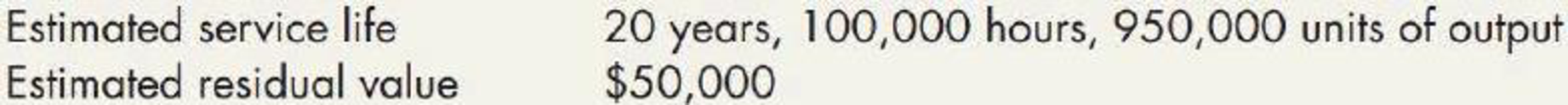Chapter 11, Problem 1P### Intermediate Accounting: Reporting...

3rd Edition
James M. Wahlen + 2 others
ISBN: 9781337788281

#### Solutions

Chapter
Section### Intermediate Accounting: Reporting...

3rd Edition
James M. Wahlen + 2 others
ISBN: 9781337788281
Textbook Problem
87 views

# Depreciation Methods Winsey Company purchased equipment on January 2, 2019, for \$700,000. The equipment has the following characteristics:During 2019 and 2020, the company used the machine for 4,500 and 5,500 hours, respectively, and produced 40,000 and 60,000 units, respectively.Required:Compute depreciation expense for 2019 and 2020 under each of the following methods: 1. straight-line method 2. activity method based on hours worked (round the depreciation rate per hour to 2 decimal places) 3. activity method based on units of output (round the depreciation rate per unit to 2 decimal places) 4. sum-of-the-years’-digits method 5. double-de dining-balance method 6. 150%-declining-balance method 7. Next Level If Winsey used a service life of 16 years, 80,000 hours, or 750,000 units of output, what would be the effect on depreciation expense under the straight-line, sum-of-the-years’-digits, and declining-balance depreciation methods? Round your answers to the nearest dollar.

1.

To determine

Calculate the depreciation expense of Company W for 2019 and 2020 under straight line method.

Explanation

Depreciation expense: Depreciation expense is a non-cash expense, which is recorded on the income statement reflecting the consumption of economic benefits of long-term asset on account of its wear and tear or obsolesces.

Straight-line depreciation method: The depreciation method which assumes that the consumption of economic benefits of long-term asset could be distributed equally throughout the useful life of the asset is referred to as straight-line method.

Calculate the depreciation expense of Company W under straight line method as follows:

Deprec

2.

To determine

Calculate the depreciation expense of Company W for 2019 and 2020 under activity method based on hours worked.

3.

To determine

Calculate the depreciation expense of Company W for 2019 and 2020 under activity method based on units of output.

4.

To determine

Calculate the depreciation expense of Company W for 2019 and 2020 under sum-of years’ digit method.

5.

To determine

Calculate the depreciation expense of Company W for 2019 and 2020 under the double-declining balance method.

6.

To determine

Calculate the depreciation expense of Company W for 2019 and 2020 under the 150%-declining balance method.

7.

To determine

Identify the effect of the depreciation expense under the straight line, sum of the year’s digit, and declining balance methods, assume Company W used a service life of 16 years, 80,000 hours, or 75,000 units of output.

### Still sussing out bartleby?

Check out a sample textbook solution.

See a sample solution

#### The Solution to Your Study Problems

Bartleby provides explanations to thousands of textbook problems written by our experts, many with advanced degrees!

Get Started

#### Why is productivity important?

Principles of Microeconomics (MindTap Course List)

#### In what ways is economics a science?

Essentials of Economics (MindTap Course List)

#### What does it mean when it is said that the United States is running a trade deficit? What impact will a trade d...

Fundamentals of Financial Management, Concise Edition (with Thomson ONE - Business School Edition, 1 term (6 months) Printed Access Card) (MindTap Course List)